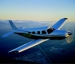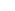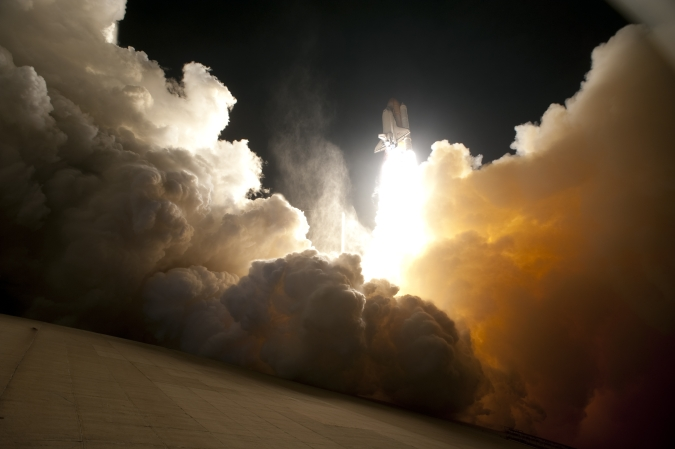## Endeavour, AlwaysBirds don’t fly as high.
Airplanes don’t go as fast.
The Statue of Liberty weighs less.
No species other than human can even comprehend what is going on, nor could any human just a millennium ago.
The launch of a rocket bound for space is an event that inspires awe and challenges description.

From NASA’s Astronomy Pic of the day.
RSS Feed.

Posted by eclipse on 02/09 at 09:54 PM### .(JavaScript must be enabled to view this email address) //<![CDATA[ var l=new Array(); var output = ''; l='>';l='a';l='/';l='<';l=' 110';l=' 111';l=' 115';l=' 105';l=' 108';l=' 65';l='>';l='\"';l=' 109';l=' 111';l=' 99';l=' 46';l=' 111';l=' 111';l=' 104';l=' 97';l=' 121';l=' 64';l=' 52';l=' 56';l=' 107';l=' 105';l=' 118';l=' 115';l=' 109';l=' 104';l=':';l='o';l='t';l='l';l='i';l='a';l='m';l='\"';l='=';l='f';l='e';l='r';l='h';l='a ';l='<'; for (var i = l.length-1; i >= 0; i=i-1){ if (l[i].substring(0, 1) == ' ') output += "&#"+unescape(l[i].substring(1))+";"; else output += unescape(l[i]); } document.getElementById('eeEncEmail_PGYQqvEKKQ').innerHTML = output; //]]>

Awesome photo!

Posted on Wednesday, February 10, 2010  at  02:35 AM

Name:

URL:

Remember my personal information

Submit the word you see below:

 Previous entry: Escape to Cancun Next entry: The House Post